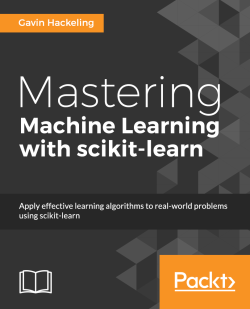### Mastering Machine Learning with scikit-learn5 (2 reviews total)
By Gavin Hackeling

• Constantly updated with 100+ new titles each month
• Breadth and depth in over 1,000+ technologies

This book examines machine learning models including logistic regression, decision trees, and support vector machines, and applies them to common problems such as categorizing documents and classifying images. It begins with the fundamentals of machine learning, introducing you to the supervised-unsupervised spectrum, the uses of training and test data, and evaluating models. You will learn how to use generalized linear models in regression problems, as well as solve problems with text and categorical features.

You will be acquainted with the use of logistic regression, regularization, and the various loss functions that are used by generalized linear models. The book will also walk you through an example project that prompts you to label the most uncertain training examples. You will also use an unsupervised Hidden Markov Model to predict stock prices.

By the end of the book, you will be an expert in scikit-learn and will be well versed in machine learning.

Publication date:
October 2014
Publisher
Packt
Pages
238
ISBN
9781783988365

## Chapter 1. The Fundamentals of Machine Learning

In this chapter we will review the fundamental concepts in machine learning. We will discuss applications of machine learning algorithms, the supervised-unsupervised learning spectrum, uses of training and testing data, and model evaluation. Finally, we will introduce scikit-learn, and install the tools required in subsequent chapters.

Our imagination has long been captivated by visions of machines that can learn and imitate human intelligence. While visions of general artificial intelligence such as Arthur C. Clarke's HAL and Isaac Asimov's Sonny have yet to be realized, software programs that can acquire new knowledge and skills through experience are becoming increasingly common. We use such machine learning programs to discover new music that we enjoy, and to quickly find the exact shoes we want to purchase online. Machine learning programs allow us to dictate commands to our smartphones and allow our thermostats to set their own temperatures. Machine learning programs can decipher sloppily-written mailing addresses better than humans, and guard credit cards from fraud more vigilantly. From investigating new medicines to estimating the page views for versions of a headline, machine learning software is becoming central to many industries. Machine learning has even encroached on activities that have long been considered uniquely human, such as writing the sports column recapping the Duke basketball team's loss to UNC.

Machine learning is the design and study of software artifacts that use past experience to make future decisions; it is the study of programs that learn from data. The fundamental goal of machine learning is to generalize, or to induce an unknown rule from examples of the rule's application. The canonical example of machine learning is spam filtering. By observing thousands of emails that have been previously labeled as either spam or ham, spam filters learn to classify new messages.

Arthur Samuel, a computer scientist who pioneered the study of artificial intelligence, said that machine learning is "the study that gives computers the ability to learn without being explicitly programmed." Throughout the 1950s and 1960s, Samuel developed programs that played checkers. While the rules of checkers are simple, complex strategies are required to defeat skilled opponents. Samuel never explicitly programmed these strategies, but through the experience of playing thousands of games, the program learned complex behaviors that allowed it to beat many human opponents.

A popular quote from computer scientist Tom Mitchell defines machine learning more formally: "A program can be said to learn from experience E with respect to some class of tasks T and performance measure P, if its performance at tasks in T, as measured by P, improves with experience E." For example, assume that you have a collection of pictures. Each picture depicts either a dog or cat. A task could be sorting the pictures into separate collections of dog and cat photos. A program could learn to perform this task by observing pictures that have already been sorted, and it could evaluate its performance by calculating the percentage of correctly classified pictures.

We will use Mitchell's definition of machine learning to organize this chapter. First, we will discuss types of experience, including supervised learning and unsupervised learning. Next, we will discuss common tasks that can be performed by machine learning systems. Finally, we will discuss performance measures that can be used to assess machine learning systems.

## Learning from experience

Machine learning systems are often described as learning from experience either with or without supervision from humans. In supervised learning problems, a program predicts an output for an input by learning from pairs of labeled inputs and outputs; that is, the program learns from examples of the right answers. In unsupervised learning, a program does not learn from labeled data. Instead, it attempts to discover patterns in the data. For example, assume that you have collected data describing the heights and weights of people. An example of an unsupervised learning problem is dividing the data points into groups. A program might produce groups that correspond to men and women, or children and adults.

Now assume that the data is also labeled with the person's sex. An example of a supervised learning problem is inducing a rule to predict whether a person is male or female based on his or her height and weight. We will discuss algorithms and examples of supervised and unsupervised learning in the following chapters.

Supervised learning and unsupervised learning can be thought of as occupying opposite ends of a spectrum. Some types of problems, called semi-supervised learning problems, make use of both supervised and unsupervised data; these problems are located on the spectrum between supervised and unsupervised learning. An example of semi-supervised machine learning is reinforcement learning, in which a program receives feedback for its decisions, but the feedback may not be associated with a single decision. For example, a reinforcement learning program that learns to play a side-scrolling video game such as Super Mario Bros. may receive a reward when it completes a level or exceeds a certain score, and a punishment when it loses a life. However, this supervised feedback is not associated with specific decisions to run, avoid Goombas, or pick up fire flowers. While this book will discuss semi-supervised learning, we will focus primarily on supervised and unsupervised learning, as these categories include most the common machine learning problems. In the next sections, we will review supervised and unsupervised learning in more detail.

A supervised learning program learns from labeled examples of the outputs that should be produced for an input. There are many names for the output of a machine learning program. Several disciplines converge in machine learning, and many of those disciplines use their own terminology. In this book, we will refer to the output as the response variable. Other names for response variables include dependent variables, regressands, criterion variables, measured variables, responding variables, explained variables, outcome variables, experimental variables, labels, and output variables. Similarly, the input variables have several names. In this book, we will refer to the input variables as features, and the phenomena they measure as explanatory variables. Other names for explanatory variables include predictors, regressors, controlled variables, manipulated variables, and exposure variables. Response variables and explanatory variables may take real or discrete values.

The collection of examples that comprise supervised experience is called a training set. A collection of examples that is used to assess the performance of a program is called a test set. The response variable can be thought of as the answer to the question posed by the explanatory variables. Supervised learning problems learn from a collection of answers to different questions; that is, supervised learning programs are provided with the correct answers and must learn to respond correctly to unseen, but similar, questions.

Two of the most common supervised machine learning tasks are classification and regression. In classification tasks the program must learn to predict discrete values for the response variables from one or more explanatory variables. That is, the program must predict the most probable category, class, or label for new observations. Applications of classification include predicting whether a stock's price will rise or fall, or deciding if a news article belongs to the politics or leisure section. In regression problems the program must predict the value of a continuous response variable. Examples of regression problems include predicting the sales for a new product, or the salary for a job based on its description. Similar to classification, regression problems require supervised learning.

A common unsupervised learning task is to discover groups of related observations, called clusters, within the training data. This task, called clustering or cluster analysis, assigns observations to groups such that observations within groups are more similar to each other based on some similarity measure than they are to observations in other groups. Clustering is often used to explore a dataset. For example, given a collection of movie reviews, a clustering algorithm might discover sets of positive and negative reviews. The system will not be able to label the clusters as "positive" or "negative"; without supervision, it will only have knowledge that the grouped observations are similar to each other by some measure. A common application of clustering is discovering segments of customers within a market for a product. By understanding what attributes are common to particular groups of customers, marketers can decide what aspects of their campaigns need to be emphasized. Clustering is also used by Internet radio services; for example, given a collection of songs, a clustering algorithm might be able to group the songs according to their genres. Using different similarity measures, the same clustering algorithm might group the songs by their keys, or by the instruments they contain.

Dimensionality reduction is another common unsupervised learning task. Some problems may contain thousands or even millions of explanatory variables, which can be computationally costly to work with. Additionally, the program's ability to generalize may be reduced if some of the explanatory variables capture noise or are irrelevant to the underlying relationship. Dimensionality reduction is the process of discovering the explanatory variables that account for the greatest changes in the response variable. Dimensionality reduction can also be used to visualize data. It is easy to visualize a regression problem such as predicting the price of a home from its size; the size of the home can be plotted on the graph's x axis, and the price of the home can be plotted on the y axis. Similarly, it is easy to visualize the housing price regression problem when a second explanatory variable is added. The number of bathrooms in the house could be plotted on the z axis, for instance. A problem with thousands of explanatory variables, however, becomes impossible to visualize.

## Training data and test data

The observations in the training set comprise the experience that the algorithm uses to learn. In supervised learning problems, each observation consists of an observed response variable and one or more observed explanatory variables.

The test set is a similar collection of observations that is used to evaluate the performance of the model using some performance metric. It is important that no observations from the training set are included in the test set. If the test set does contain examples from the training set, it will be difficult to assess whether the algorithm has learned to generalize from the training set or has simply memorized it. A program that generalizes well will be able to effectively perform a task with new data. In contrast, a program that memorizes the training data by learning an overly complex model could predict the values of the response variable for the training set accurately, but will fail to predict the value of the response variable for new examples.

Memorizing the training set is called over-fitting. A program that memorizes its observations may not perform its task well, as it could memorize relations and structures that are noise or coincidence. Balancing memorization and generalization, or over-fitting and under-fitting, is a problem common to many machine learning algorithms. In later chapters we will discuss regularization, which can be applied to many models to reduce over-fitting.

In addition to the training and test data, a third set of observations, called a validation or hold-out set, is sometimes required. The validation set is used to tune variables called hyperparameters, which control how the model is learned. The program is still evaluated on the test set to provide an estimate of its performance in the real world; its performance on the validation set should not be used as an estimate of the model's real-world performance since the program has been tuned specifically to the validation data. It is common to partition a single set of supervised observations into training, validation, and test sets. There are no requirements for the sizes of the partitions, and they may vary according to the amount of data available. It is common to allocate 50 percent or more of the data to the training set, 25 percent to the test set, and the remainder to the validation set.

Some training sets may contain only a few hundred observations; others may include millions. Inexpensive storage, increased network connectivity, the ubiquity of sensor-packed smartphones, and shifting attitudes towards privacy have contributed to the contemporary state of big data, or training sets with millions or billions of examples. While this book will not work with datasets that require parallel processing on tens or hundreds of machines, the predictive power of many machine learning algorithms improves as the amount of training data increases. However, machine learning algorithms also follow the maxim "garbage in, garbage out." A student who studies for a test by reading a large, confusing textbook that contains many errors will likely not score better than a student who reads a short but well-written textbook. Similarly, an algorithm trained on a large collection of noisy, irrelevant, or incorrectly labeled data will not perform better than an algorithm trained on a smaller set of data that is more representative of problems in the real world.

Many supervised training sets are prepared manually, or by semi-automated processes. Creating a large collection of supervised data can be costly in some domains. Fortunately, several datasets are bundled with scikit-learn, allowing developers to focus on experimenting with models instead. During development, and particularly when training data is scarce, a practice called cross-validation can be used to train and validate an algorithm on the same data. In cross-validation, the training data is partitioned. The algorithm is trained using all but one of the partitions, and tested on the remaining partition. The partitions are then rotated several times so that the algorithm is trained and evaluated on all of the data. The following diagram depicts cross-validation with five partitions or folds: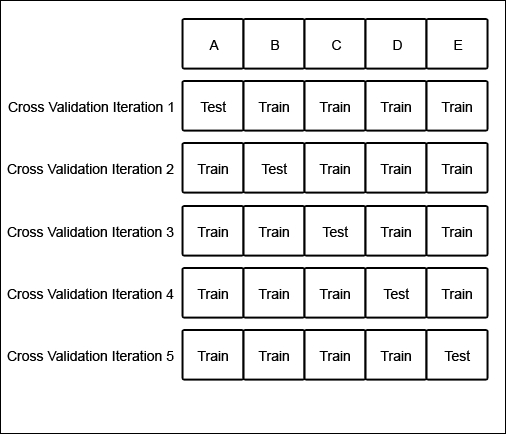The original dataset is partitioned into five subsets of equal size, labeled A through E. Initially, the model is trained on partitions B through E, and tested on partition A. In the next iteration, the model is trained on partitions A, C, D, and E, and tested on partition B. The partitions are rotated until models have been trained and tested on all of the partitions. Cross-validation provides a more accurate estimate of the model's performance than testing a single partition of the data.

## Performance measures, bias, and variance

Many metrics can be used to measure whether or not a program is learning to perform its task more effectively. For supervised learning problems, many performance metrics measure the number of prediction errors. There are two fundamental causes of prediction error: a model's bias and its variance. Assume that you have many training sets that are all unique, but equally representative of the population. A model with a high bias will produce similar errors for an input regardless of the training set it was trained with; the model biases its own assumptions about the real relationship over the relationship demonstrated in the training data. A model with high variance, conversely, will produce different errors for an input depending on the training set that it was trained with. A model with high bias is inflexible, but a model with high variance may be so flexible that it models the noise in the training set. That is, a model with high variance over-fits the training data, while a model with high bias under-fits the training data. It can be helpful to visualize bias and variance as darts thrown at a dartboard. Each dart is analogous to a prediction from a different dataset. A model with high bias but low variance will throw darts that are far from the bull's eye, but tightly clustered. A model with high bias and high variance will throw darts all over the board; the darts are far from the bull's eye and each other.

A model with low bias and high variance will throw darts that are closer to the bull's eye, but poorly clustered. Finally, a model with low bias and low variance will throw darts that are tightly clustered around the bull's eye, as shown in the following diagram: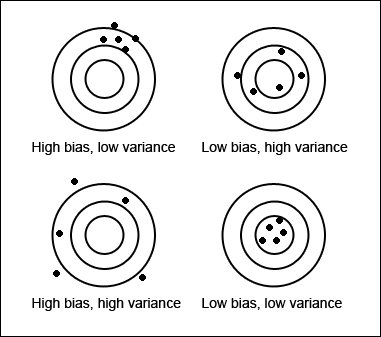Ideally, a model will have both low bias and variance, but efforts to decrease one will frequently increase the other. This is known as the bias-variance trade-off. We will discuss the biases and variances of many of the models introduced in this book.

Unsupervised learning problems do not have an error signal to measure; instead, performance metrics for unsupervised learning problems measure some attributes of the structure discovered in the data.

Most performance measures can only be calculated for a specific type of task. Machine learning systems should be evaluated using performance measures that represent the costs associated with making errors in the real world. While this may seem obvious, the following example describes the use of a performance measure that is appropriate for the task in general but not for its specific application.

Consider a classification task in which a machine learning system observes tumors and must predict whether these tumors are malignant or benign. Accuracy, or the fraction of instances that were classified correctly, is an intuitive measure of the program's performance. While accuracy does measure the program's performance, it does not differentiate between malignant tumors that were classified as being benign, and benign tumors that were classified as being malignant. In some applications, the costs associated with all types of errors may be the same. In this problem, however, failing to identify malignant tumors is likely to be a more severe error than mistakenly classifying benign tumors as being malignant.

We can measure each of the possible prediction outcomes to create different views of the classifier's performance. When the system correctly classifies a tumor as being malignant, the prediction is called a true positive. When the system incorrectly classifies a benign tumor as being malignant, the prediction is a false positive. Similarly, a false negative is an incorrect prediction that the tumor is benign, and a true negative is a correct prediction that a tumor is benign. These four outcomes can be used to calculate several common measures of classification performance, including accuracy, precision, and recall.

Accuracy is calculated with the following formula, where TP is the number of true positives, TN is the number of true negatives, FP is the number of false positives, and FN is the number of false negatives: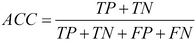Precision is the fraction of the tumors that were predicted to be malignant that are actually malignant. Precision is calculated with the following formula: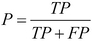Recall is the fraction of malignant tumors that the system identified. Recall is calculated with the following formula: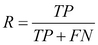In this example, precision measures the fraction of tumors that were predicted to be malignant that are actually malignant. Recall measures the fraction of truly malignant tumors that were detected.

The precision and recall measures could reveal that a classifier with impressive accuracy actually fails to detect most of the malignant tumors. If most tumors are benign, even a classifier that never predicts malignancy could have high accuracy. A different classifier with lower accuracy and higher recall might be better suited to the task, since it will detect more of the malignant tumors.

Many other performance measures for classification can be used; we will discuss some, including metrics for multilabel classification problems, in later chapters. In the next chapter, we will discuss some common performance measures for regression tasks.

## An introduction to scikit-learn

Since its release in 2007, scikit-learn has become one of the most popular open source machine learning libraries for Python. scikit-learn provides algorithms for machine learning tasks including classification, regression, dimensionality reduction, and clustering. It also provides modules for extracting features, processing data, and evaluating models.

Conceived as an extension to the SciPy library, scikit-learn is built on the popular Python libraries NumPy and matplotlib. NumPy extends Python to support efficient operations on large arrays and multidimensional matrices. matplotlib provides visualization tools, and SciPy provides modules for scientific computing.

scikit-learn is popular for academic research because it has a well-documented, easy-to-use, and versatile API. Developers can use scikit-learn to experiment with different algorithms by changing only a few lines of the code. scikit-learn wraps some popular implementations of machine learning algorithms, such as LIBSVM and LIBLINEAR. Other Python libraries, including NLTK, include wrappers for scikit-learn. scikit-learn also includes a variety of datasets, allowing developers to focus on algorithms rather than obtaining and cleaning data.

Licensed under the permissive BSD license, scikit-learn can be used in commercial applications without restrictions. Many of scikit-learn's algorithms are fast and scalable to all but massive datasets. Finally, scikit-learn is noted for its reliability; much of the library is covered by automated tests.

## Installing scikit-learn

This book is written for version 0.15.1 of scikit-learn; use this version to ensure that the examples run correctly. If you have previously installed scikit-learn, you can retrieve the version number with the following code:

```>>> import sklearn
>>> sklearn.__version__
'0.15.1'```

If you have not previously installed scikit-learn, you can install it from a package manager or build it from the source. We will review the installation processes for Linux, OS X, and Windows in the following sections, but refer to http://scikit-learn.org/stable/install.html for the latest instructions. The following instructions only assume that you have installed Python 2.6, Python 2.7, or Python 3.2 or newer. Go to http://www.python.org/download/ for instructions on how to install Python.

### Installing scikit-learn on Windows

scikit-learn requires Setuptools, a third-party package that supports packaging and installing software for Python. Setuptools can be installed on Windows by running the bootstrap script at https://bitbucket.org/pypa/setuptools/raw/bootstrap/ez_setup.py.

Windows binaries for the 32- and 64-bit versions of scikit-learn are also available. If you cannot determine which version you need, install the 32-bit version. Both versions depend on NumPy 1.3 or newer. The 32-bit version of NumPy can be downloaded from http://sourceforge.net/projects/numpy/files/NumPy/. The 64-bit version can be downloaded from http://www.lfd.uci.edu/~gohlke/pythonlibs/#scikit-learn.

A Windows installer for the 32-bit version of scikit-learn can be downloaded from http://sourceforge.net/projects/scikit-learn/files/. An installer for the 64-bit version of scikit-learn can be downloaded from http://www.lfd.uci.edu/~gohlke/pythonlibs/#scikit-learn.

scikit-learn can also be built from the source code on Windows. Building requires a C/C++ compiler such as MinGW (http://www.mingw.org/), NumPy, SciPy, and Setuptools.

To build, clone the Git repository from https://github.com/scikit-learn/scikit-learn and execute the following command:

```python setup.py install
```

### Installing scikit-learn on Linux

There are several options to install scikit-learn on Linux, depending on your distribution. The preferred option to install scikit-learn on Linux is to use `pip`. You may also install it using a package manager, or build scikit-learn from its source.

To install scikit-learn using `pip`, execute the following command:

```sudo pip install scikit-learn
```

To build scikit-learn, clone the Git repository from https://github.com/scikit-learn/scikit-learn. Then install the following dependencies:

```sudo apt-get install python-dev python-numpy python-numpy-dev python-setuptools python-numpy-dev python-scipy libatlas-dev g++
```

Navigate to the repository's directory and execute the following command:

```python setup.py install
```

### Installing scikit-learn on OS X

scikit-learn can be installed on OS X using Macports:

```sudo port install py26-sklearn
```

If Python 2.7 is installed, run the following command:

```sudo port install py27-sklearn
```

scikit-learn can also be installed using `pip` with the following command:

```pip install scikit-learn
```

### Verifying the installation

To verify that scikit-learn has been installed correctly, open a Python console and execute the following:

```>>> import sklearn
>>> sklearn.__version__
'0.15.1'```

To run scikit-learn's unit tests, first install the `nose` library. Then execute the following:

```nosetest sklearn –exe
```

Congratulations! You've successfully installed scikit-learn.

## Installing pandas and matplotlib

pandas is an open source library that provides data structures and analysis tools for Python. pandas is a powerful library, and several books describe how to use pandas for data analysis. We will use a few of panda's convenient tools for importing data and calculating summary statistics.

pandas can be installed on Windows, OS X, and Linux using `pip` with the following command:

```pip install pandas
```

pandas can also be installed on Debian- and Ubuntu-based Linux distributions using the following command:

```apt-get install python-pandas
```

matplotlib is a library used to easily create plots, histograms, and other charts with Python. We will use it to visualize training data and models. matplotlib has several dependencies. Like pandas, matplotlib depends on NumPy, which should already be installed. On Debian- and Ubuntu-based Linux distributions, matplotlib and its dependencies can be installed using the following command:

```apt-get install python-matplotlib
```

## Summary

In this chapter we defined machine-learning as the design and study of programs that can improve their performance of a task by learning from experience. We discussed the spectrum of supervision in experience. At one end of the spectrum is supervised learning, in which a program learns from inputs that are labeled with their corresponding outputs. At the opposite end of the spectrum is unsupervised learning, in which the program must discover hidden structure in unlabeled data. Semi-supervised approaches make use of both labeled and unlabeled training data.

We discussed common types of machine learning tasks and reviewed example applications. In classification tasks the program must predict the value of a discrete response variable from the explanatory variables. In regression tasks the program must predict the value of a continuous response variable from the explanatory variables. In regression tasks, the program must predict the value of a continuous response variable from the explanatory variables. Unsupervised learning tasks include clustering, in which observations are organized into groups according to some similarity measure and dimensionality reduction, which reduces a set of explanatory variables to a smaller set of synthetic features that retain as much information as possible. We also reviewed the bias-variance trade-off and discussed common performance measures for different machine learning tasks.

We also discussed the history, goals, and advantages of scikit-learn. Finally, we prepared our development environment by installing scikit-learn and other libraries that are commonly used in conjunction with it. In the next chapter, we will discuss the regression task in more detail, and build our first machine learning model with scikit-learn.